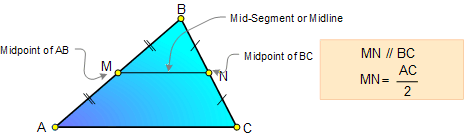# Geometry Problem 89. Area of Triangle and Quadrilateral, Midpoints of Diagonals, Median of a triangle. High School, College, Math Education

 In the figure below, given a triangle AED, M and N are the midpoints of cevians AC and DB respectively. If S1, S2, and S3 are the areas of the triangles EBM, ECN, and BEC respectively, prove that:.HINTS: 1. CEVIAN: A Cevian is a line segment which joins a vertex of a triangle with a point on the opposite side (or its extension). 2. AREA OF A TRIANGLE: Median Area Fact: A median divides the triangle into two triangles of equal area.3. Mid-Segment or Midline of a Triangle Theorem: If a line MN joins the midpoints of two sides of a triangle, then it is parallel to the third side and its length is one-half the length of the third side.Home | Search | Geometry | Problems | All Problems | Open Problems | Visual Index | Art | 81-90 | Email | View or post a solution